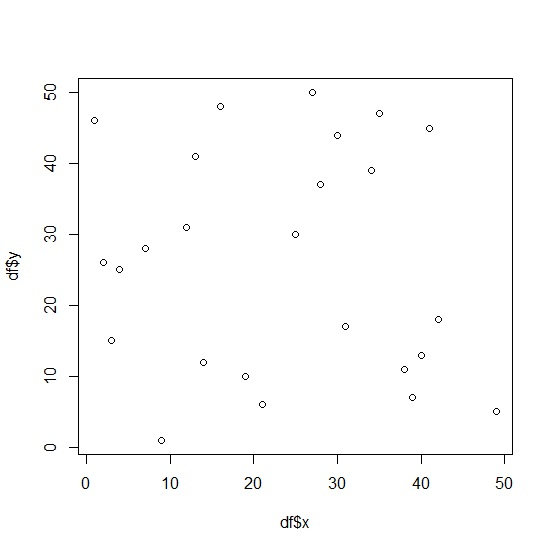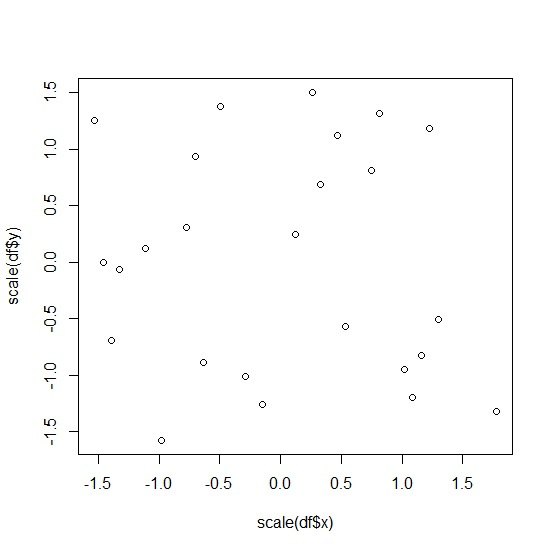# How to create scatterplot by standardizing the columns of a data frame in base R?

R ProgrammingServer Side ProgrammingProgramming

To create scatterplot by standardizing the columns of a data frame in base R, we can follow the below step −

• First of all, create a data frame.

• Then, create scatterplot between two columns with their default value.

• After that, create the scatterplot with scale function.

## Create the data frame

Let’s create a data frame as shown below −

Live Demo

x<-sample(1:50,25)
y<=-sample(1:50,25)
df<-data.frame(x,y)
df

On executing, the above script generates the below output(this output will vary on your system due to randomization) −

## Output

  x   y
1 42 18
2 9   1
3 16 48
4 49  5
5 25 30
6 2  26
7 3  15
8 13 41
9 12 31
10 1 46
11 35 47
12 34 39
13 38 11
14 4 25
15 30 44
16 41 45
17 27 50
18 21 6
19 40 13
20 31 17
21 19 10
22 14 12
23 28 37
24 39 7
25 7 28

## Create the scatterplot

Using plot function to create the scatterplot between x and y −

Live Demo

x<-sample(1:50,25)
y<-sample(1:50,25)
df<-data.frame(x,y)
plot(df$x,df$y)

## Output## Create the scatterplot after standardizing the columns

Using scale function to create the scatterplot between standardized values of x and y −

Live Demo

x<-sample(1:50,25)
y<-sample(1:50,25)
df<-data.frame(x,y)
plot(scale(df$x),scale(df$y))

## Output